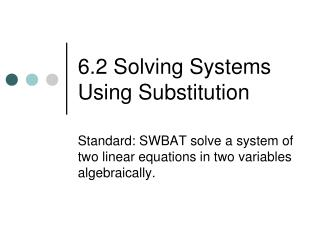DownloadDownload Presentation6.2 Solving Systems Using Substitution

# 6.2 Solving Systems Using Substitution

Télécharger la présentation## 6.2 Solving Systems Using Substitution

- - - - - - - - - - - - - - - - - - - - - - - - - - - E N D - - - - - - - - - - - - - - - - - - - - - - - - - - -
##### Presentation Transcript

1. 6.2 Solving Systems Using Substitution Standard: SWBAT solve a system of two linear equations in two variables algebraically.

2. Mini Quiz 50 • Is (4, 0) a solution of the system of equations? 2x + y = 8 • -x + 3y = 4 • Solve the System of Equation by graphing: • 2y = 4x • -y = -2x

3. Quick Review • Solve the equation for x: 2y + x =12 • x = -2y + 12 • How does the graph look when there is “no solution”? • The lines are parallel and never intersect. • How does the graph look when there are “infinitely many solutions”? • The two lines are the same line.

4. Solving by Substitution • Pick an equation (that’s easiest to get one of the variables by itself) and solve for a variable • Substitute into the OTHER equation • Solve for the first variable • Substitute and solve for the second variable (doesn’t matter which equation) • Check your solution (YES, you need to check your answer!)

5. Pick Equation • Substitute • Solve • Substitute and Solve • Check Solving by Substitution 1. Solve the linear system -x + y = 4 -8x + 2y = 2 -x + y = 4 +x +x y = x + 4 Check ( 1, 5) -x + y = 4 -1 + 5 = 4 4 = 4  -8x + 2y = 2 -8(1) + 2(5) = 2 -8 + 10 = 2 2 = 2  -8x + 2(x + 4) = 2 -8x + 2x + 8 = 2 -6x + 8 = 2 -6x = -6 x = 1 -x + y = 4 -(1) + y = 4 y = 5 (1, 5)

6. Pick Equation • Substitute • Solve • Substitute and Solve • Check Substitution Solve the linear system 2. y = 2x – 4 x = y + 3 3. –m + n = 1 2m + n = -2 4. 2c + 2d = 3 c – 4d = -1 ( 1, -2) (-1, 0) (1, ½)

7. Application 5. Your school must transport 193 people to a competition. There are eight drivers available and two types of vehicles. The school buses seat 51 people each, and the minivans seat 8 people each. How many buses and minivans will be needed? b = number of school buses m = number of mini vans b + m = 8 51b + 8m = 193 (3, 5)

8. Substitution – No solution/Infinite solutions 6. y = 3x + 2 6x – 2y = -4 7. y = 2x + 6 4x – 2y = 8 8. 2x + y = -1 -6x – 3y = -15 9. 15x – 5y = -20 -3x + y = 4 Infinite Solution No Solutions No Solutions Infinite Solution

9. Wrap Up Substitution Method • Pick an equation • Substitute • Solve • Substitute and solve • Check No Solution x and y cancels and two sides are different Infinitely Many Solutions x and y cancels and both sides are equal HW: P. 284 # 1-5 odd, 9-17 odd; P. 286 #45-47 all DLUQ: What happens to the variables when you have “no solution” or “infinitely many solutions”?